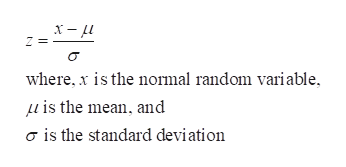# The mean amount of time it takes a kidney stone to pass is 16 days and the standard deviation is 5 days. Suppose that one individual is randomly chosen. Let X = time to pass the kidney stone.a. What is the distribution of X? X ~ N(,)b. Find the probability that a randomly selected person with a kidney stone will take longer than 14 days to pass it. Round to 4 decimal places.

Question
77 views

The mean amount of time it takes a kidney stone to pass is 16 days and the standard deviation is 5 days. Suppose that one individual is randomly chosen. Let X = time to pass the kidney stone.

a. What is the distribution of X? X ~ N(,)

b. Find the probability that a randomly selected person with a kidney stone will take longer than 14 days to pass it. Round to 4 decimal places.

check_circle

Step 1

Standard normal distribution:

The standard normal distribution is a special case of normal distribution, in which the mean of the distribution is 0 and standard deviation of the distribution is 1. That is X~N(µ, σ). The Z-scores can be calculated using the given formula.help_outlineImage Transcriptionclosewhere, x is the normal random variable is the mean, and o is the standard deviation fullscreen
Step 2

a.

In this context, it is given that the average time to pass the kidney stone is 16 days corresponding to its standard deviation of 5 days.

Denote X as the time to pass...

### Want to see the full answer?

See Solution

#### Want to see this answer and more?

Solutions are written by subject experts who are available 24/7. Questions are typically answered within 1 hour.*

See Solution
*Response times may vary by subject and question.
Tagged in

### Hypothesis Testing